Calculations of volumes, areas and circumference

Area  Circle, Sphere, Cone, Triangle. Parallelogram, Box, Trapezoid.
Volume  Sphere, Cylinder, Cone, Box, Tank with sloping sides
Other   Circumference of circle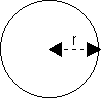Area of a circle 3.1415 x r2 Where r = radius of the circle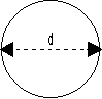Circumference of a circle 3.1415 x d Where d = diameter of the circleVolume of a sphere 4/3 x 3.1415 x r3 Where r = radius of the circleArea of a sphere 4 x 3.1415 x r2 Where r = radius of the circle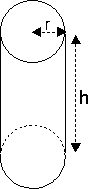Volume of a cylinder / pipe 3.1415 x r2 x h Where r = radius of the circle h = height of cylinder / pipe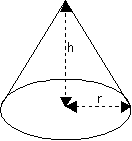Volume of a cone 1/3 x 3.1415 x r2 x h Where r = radius of the circle h = height of cone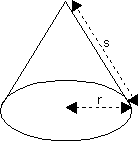Area of a cone 3.1415 x (r x s) + (3.1415 x r2) Where r = radius of the circle s = length of side of cone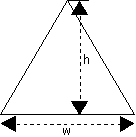Area of a triangle ( w x h ) / 2 Where w = width h = height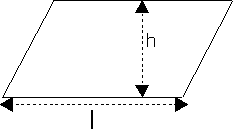Area of a parallelogram h x l Where h = height l = length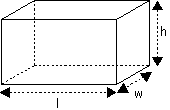Volume of a box w x l x h Where w = width h = height l = lengthArea of a box 2 x [(w x l ) + ( l x h) + (h x w)] Where w = width h = height l = length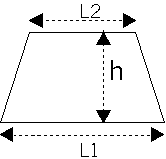Area of a trapezoid h x ((L1 + L2)/2) Where h = height L1 and L2 = top and bottom lengths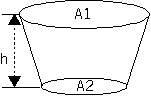Volume of a tank [(A1 + A2)/2] x h Where h = height A1 and A2 = area of top and bottom of tank